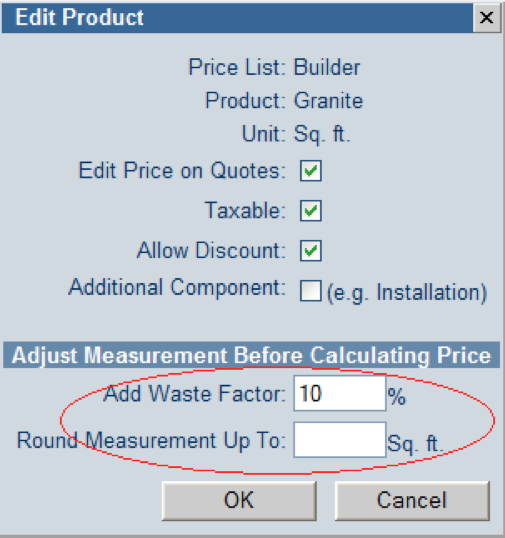You can add a waste factor and/or round a measurement up before the price on a quote is calculated.

1. Go to Quote::Edit Prices
2. In the Product column, double-click on the name of the appropriate product. Enter a Waste Factor % or Round Measurement Up To. The calculated measurements will be adjusted by these amounts in order to calculate the price. If you enter both, the waste factor % will be applied before rounding.Example 1:
Measurement = 50 sq. ft.
Waste Factor % = 10%
Round Measurement Up To: ___
Measurement used in pricing = 55 sq. ft.

Example 2:
Measurement = 51 sq. ft.
Waste Factor % ___
Round Measurement Up To: 50 sq. ft.
Measurement used in pricing = 100 sq. ft.

Example 3:
Measurement = 50 sq. ft.
Waste Factor % = 10%
Round Measurement Up To: 50 sq. ft.
Measurement used in pricing = 100 sq. ft.